Anzeige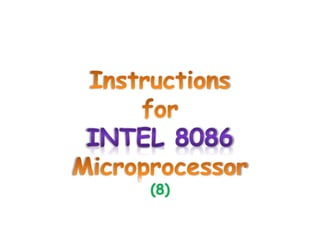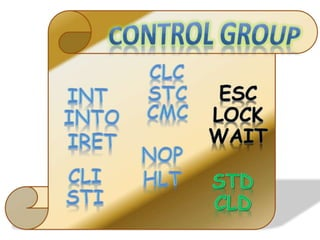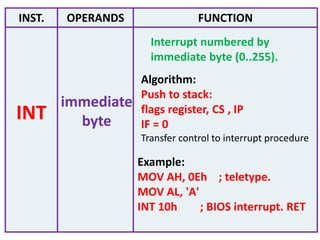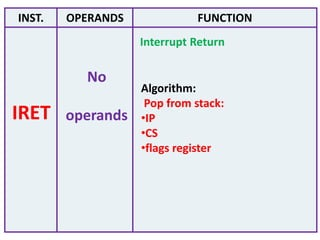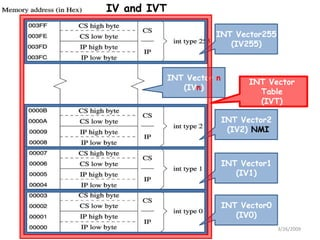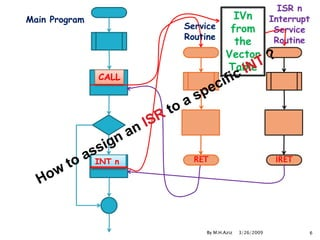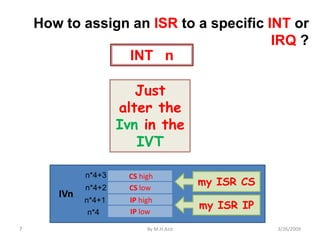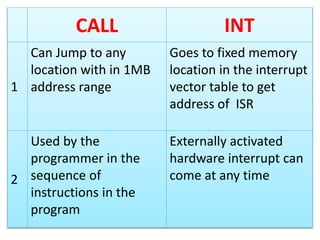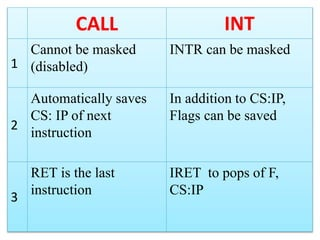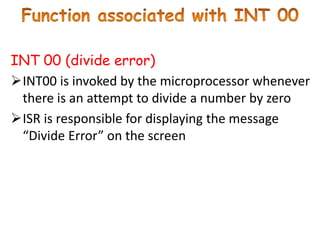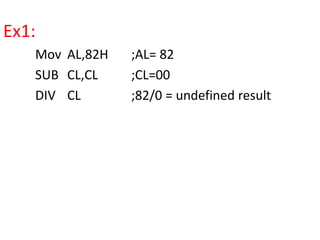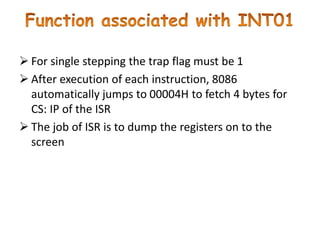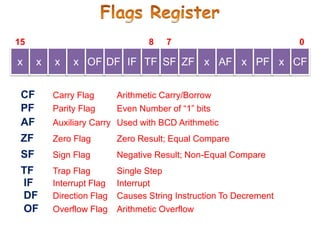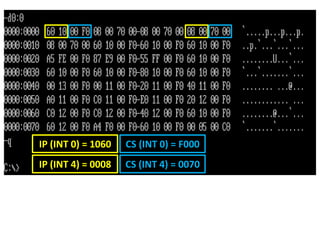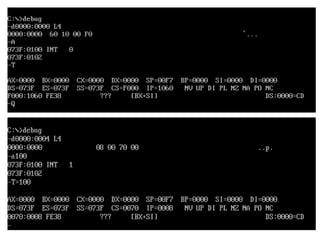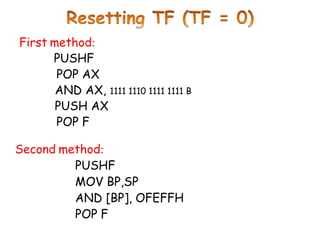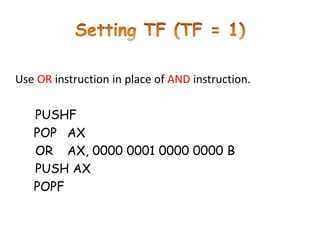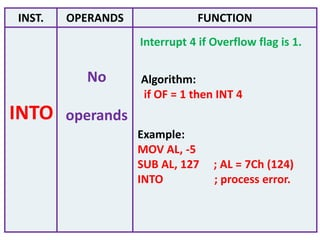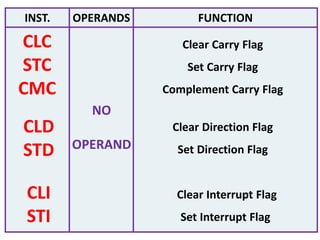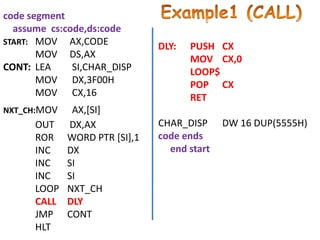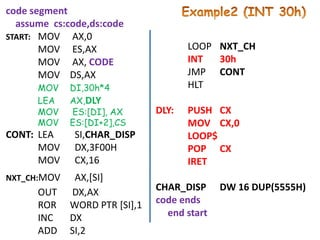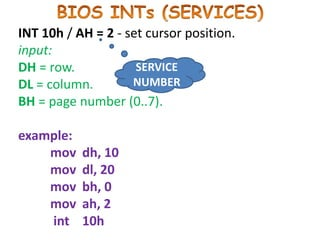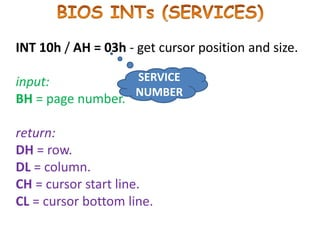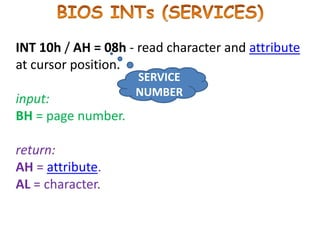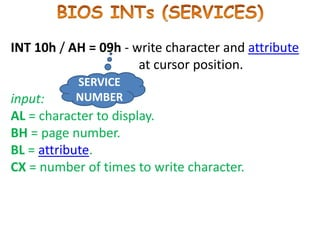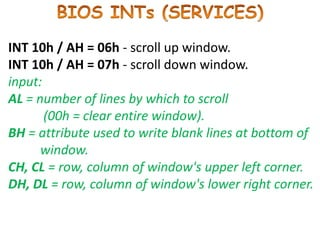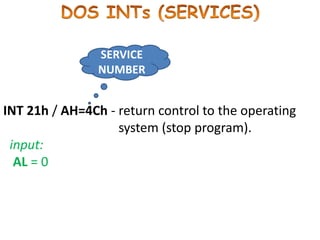1 von 28
Anzeige

### Instruction 8.pptx

1. (8)
2. INST. OPERANDS FUNCTION immediate byte Interrupt numbered by immediate byte (0..255). INT Algorithm: Push to stack: flags register, CS , IP IF = 0 Transfer control to interrupt procedure Example: MOV AH, 0Eh ; teletype. MOV AL, 'A' INT 10h ; BIOS interrupt. RET
3. INST. OPERANDS FUNCTION No operands Interrupt Return IRET Algorithm: Pop from stack: •IP •CS •flags register
4. 3/26/2009 By M.H.Aziz 5 INT Vector2 (IV2) NMI INT Vector1 (IV1) INT Vector0 (IV0) INT Vector255 (IV255) INT Vector n (IVn) INT Vector Table (IVT) IV and IVT
5. ISR n Interrupt Service Routine 3/26/2009 By M.H.Aziz 6 CALL RET Main Program INT n IRET IVn from the Vector Table Service Routine
6. Just alter the Ivn in the IVT 3/26/2009 By M.H.Aziz 7 How to assign an ISR to a specific INT or IRQ ? INT n CS high CS low IP high IP low IV2 NMI 2*4 2*4+1 2*4+2 2*4+3 CS high CS low IP high IP low IVn n*4 n*4+1 n*4+2 n*4+3 my ISR CS my ISR IP
7. CALL INT 1 Can Jump to any location with in 1MB address range Goes to fixed memory location in the interrupt vector table to get address of ISR 2 Used by the programmer in the sequence of instructions in the program Externally activated hardware interrupt can come at any time
8. CALL INT 1 Cannot be masked (disabled) INTR can be masked 2 Automatically saves CS: IP of next instruction In addition to CS:IP, Flags can be saved 3 RET is the last instruction IRET to pops of F, CS:IP
9. INT 00 (divide error) INT00 is invoked by the microprocessor whenever there is an attempt to divide a number by zero ISR is responsible for displaying the message “Divide Error” on the screen
10. Ex1: Mov AL,82H ;AL= 82 SUB CL,CL ;CL=00 DIV CL ;82/0 = undefined result
11.  For single stepping the trap flag must be 1  After execution of each instruction, 8086 automatically jumps to 00004H to fetch 4 bytes for CS: IP of the ISR  The job of ISR is to dump the registers on to the screen
12. x x x x OF DF IF TF SF ZF x AF x PF x CF 0 CF Carry Flag Arithmetic Carry/Borrow PF Parity Flag Even Number of “1” bits AF Auxiliary Carry Used with BCD Arithmetic OF Overflow Flag Arithmetic Overflow SF Sign Flag Negative Result; Non-Equal Compare ZF Zero Flag Zero Result; Equal Compare TF Trap Flag Single Step DF Direction Flag Causes String Instruction To Decrement IF Interrupt Flag Interrupt 7 8 15
13. IP (INT 0) = 1060 CS (INT 0) = F000 IP (INT 4) = 0008 CS (INT 4) = 0070
14. First method: PUSHF POP AX AND AX, 1111 1110 1111 1111 B PUSH AX POP F Second method: PUSHF MOV BP,SP AND [BP], OFEFFH POP F
15. Use OR instruction in place of AND instruction. PUSHF POP AX OR AX, 0000 0001 0000 0000 B PUSH AX POPF
16. INST. OPERANDS FUNCTION No operands Interrupt 4 if Overflow flag is 1. INTO Algorithm: if OF = 1 then INT 4 Example: MOV AL, -5 SUB AL, 127 ; AL = 7Ch (124) INTO ; process error.
17. INST. OPERANDS FUNCTION Clear Carry Flag Set Carry Flag Complement Carry Flag CLC STC CMC CLD STD Clear Direction Flag Set Direction Flag CLI STI Clear Interrupt Flag Set Interrupt Flag NO OPERAND
18. code segment assume cs:code,ds:code START: MOV AX,CODE MOV DS,AX CONT: LEA SI,CHAR_DISP MOV DX,3F00H MOV CX,16 NXT_CH:MOV AX,[SI] OUT DX,AX ROR WORD PTR [SI],1 INC DX INC SI INC SI LOOP NXT_CH CALL DLY JMP CONT HLT DLY: PUSH CX MOV CX,0 LOOP\$ POP CX RET CHAR_DISP DW 16 DUP(5555H) code ends end start
19. code segment assume cs:code,ds:code START: MOV AX,0 MOV ES,AX MOV AX, CODE MOV DS,AX MOV DI,30h*4 LEA AX,DLY MOV ES:[DI], AX MOV ES:[DI+2],CS CONT: LEA SI,CHAR_DISP MOV DX,3F00H MOV CX,16 NXT_CH:MOV AX,[SI] OUT DX,AX ROR WORD PTR [SI],1 INC DX ADD SI,2 LOOP NXT_CH INT 30h JMP CONT HLT DLY: PUSH CX MOV CX,0 LOOP\$ POP CX IRET CHAR_DISP DW 16 DUP(5555H) code ends end start
20. INT 10h / AH = 2 - set cursor position. input: DH = row. DL = column. BH = page number (0..7). example: mov dh, 10 mov dl, 20 mov bh, 0 mov ah, 2 int 10h SERVICE NUMBER
21. INT 10h / AH = 03h - get cursor position and size. input: BH = page number. return: DH = row. DL = column. CH = cursor start line. CL = cursor bottom line. SERVICE NUMBER
22. INT 10h / AH = 08h - read character and attribute at cursor position. input: BH = page number. return: AH = attribute. AL = character. SERVICE NUMBER
23. INT 10h / AH = 09h - write character and attribute at cursor position. input: AL = character to display. BH = page number. BL = attribute. CX = number of times to write character. SERVICE NUMBER
24. INT 10h / AH = 06h - scroll up window. INT 10h / AH = 07h - scroll down window. input: AL = number of lines by which to scroll (00h = clear entire window). BH = attribute used to write blank lines at bottom of window. CH, CL = row, column of window's upper left corner. DH, DL = row, column of window's lower right corner.
25. INT 21h / AH=4Ch - return control to the operating system (stop program). input: AL = 0 SERVICE NUMBER
Anzeige